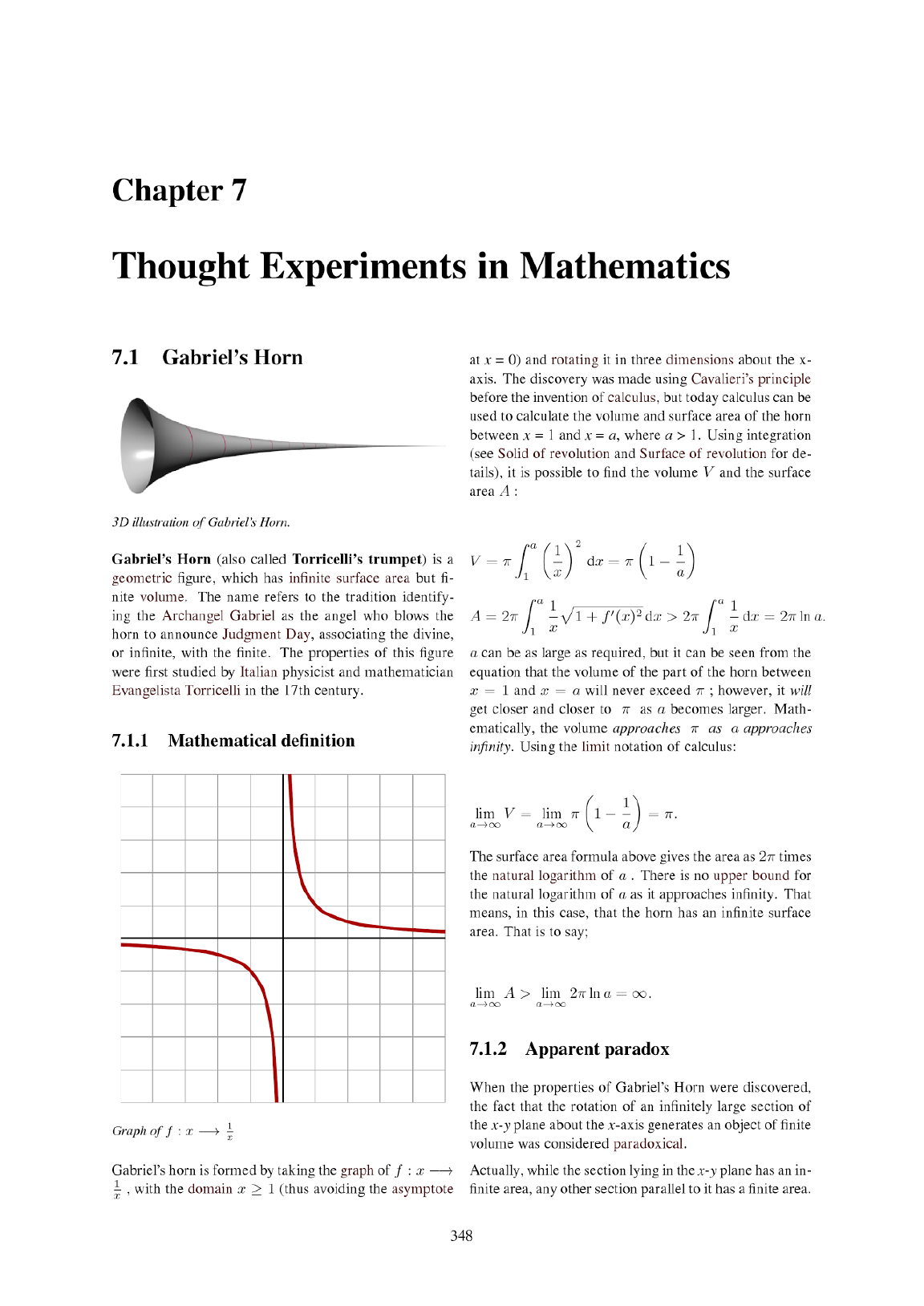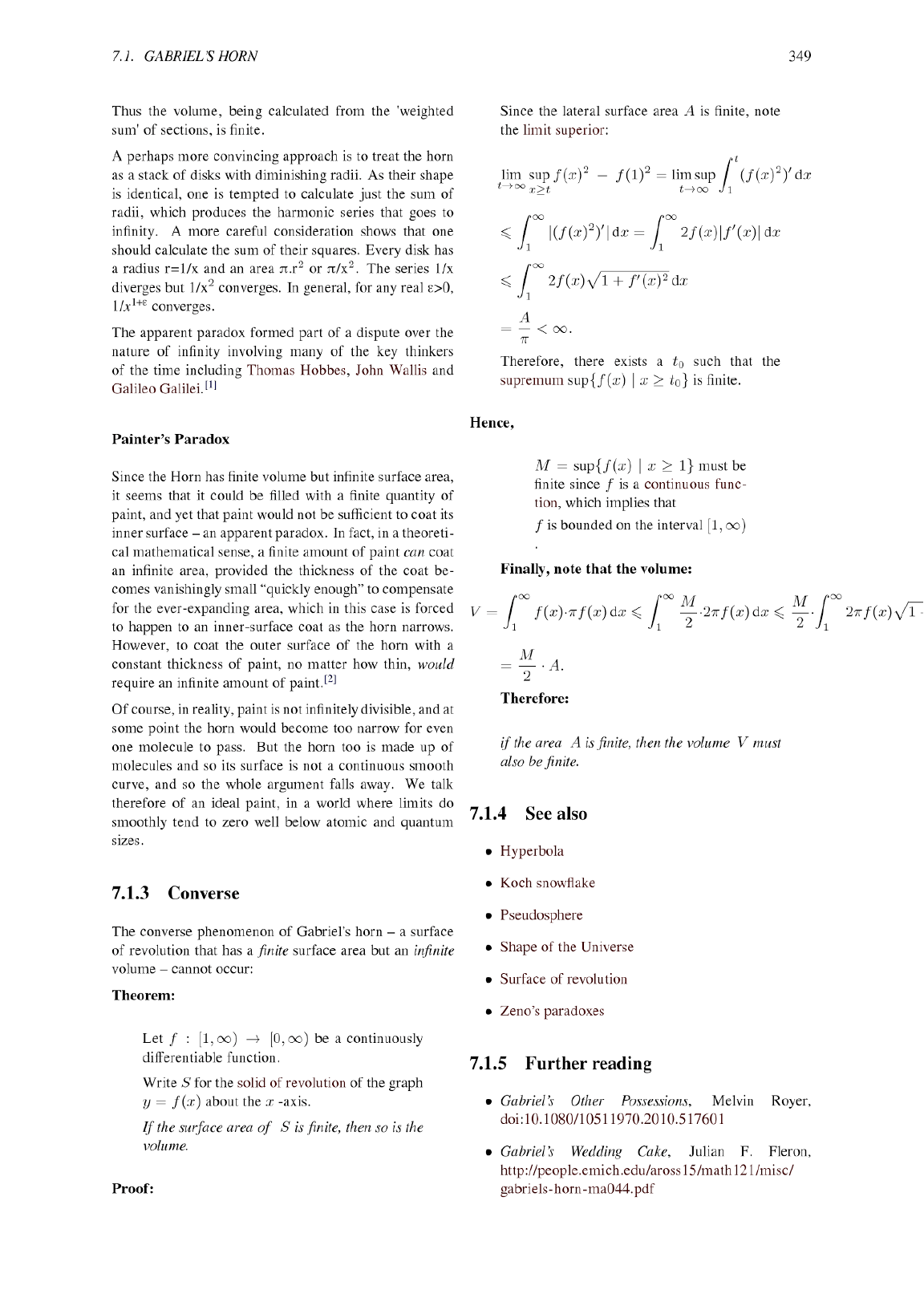*"To understand this for sense, it is not required that a man shoul...
Bonaventura Francesco Cavalieri was an Italian mathematician of the...
**Cavalieri's Principle:** states that if two objects are between t...
The volume of the horn is fairly simple to determine with mordern c...
Determining the surface of the horn is slightly more "difficult". T...
Three-dimensional illustration of Gabriel’s Horn. This horn shape h...
The section parallel to x-y plane has finite end point. Assume the ...
How this "weighted sum" is computed?
Other very interesting articles related to this topic: - [A Parado...Other very interesting articles related to this topic: - [A Paradoxical Paint Pail](http://www.maa.org/sites/default/files/lynch11200532298.pdf) - [Gabriel’s Wedding Cake](http://people.emich.edu/aross15/math121/misc/gabriels-horn-ma044.pdf) - [Mathematica - Gabriel's Horn](http://mathworld.wolfram.com/GabrielsHorn.html) How this "weighted sum" is computed? Three-dimensional illustration of Gabriel’s Horn. This horn shape has an interesting, counterintuitive property: **a finite volume but an infinite surface area.** ![GH](http://67.media.tumblr.com/47e8ed75408372defa0ccdf3ce155147/tumblr_n7umskeuNz1tzs5dao3_1280.gif) Create your own Gabriel Horn with Mathematica: mathematica x[u_, v_] := u y[u_, v_] := Cos[v]/u z[u_, v_] := Sin[v]/u Manipulate[ParametricPlot3D[{{x[u, v], y[u, v], z[u, v]}}, {u, 1, umax}, {v, 0, 2*Pi}, PlotRange -> {{0, 20}, {-1, 1}, {-1, 1}}, Mesh -> {Floor[umax], 20}, Axes -> False, Boxed -> False], {{umax, 20}, 1.1, 20}]  Source: [Fouriest Seriess.](http://fouriestseries.tumblr.com/post/90296337418/gabriels-horn-and-the-painters-paradox) The volume of the horn is fairly simple to determine with mordern calculous. We are taking the finite horn from $$x = 1, x = N$$ and then allowing N to become infinitely large, we can then write the volume of the horn: \begin{eqnarray*} V &=& \pi \int_1^N \left( \frac{1}{x} \right)^2 dx\\ &=& \pi \left [ - \frac{1}{x}\right]_1^N\\ &=& \pi \left ( 1 - \frac{1}{N}\right) \end{eqnarray*} and as it is trivial to see that as $N \rightarrow \infty$ the volume approaches the finite value of $\pi$. \begin{eqnarray*} \lim_{x\to\infty} V &=& \lim_{x\to\infty} \left ( 1 - \frac{1}{x}\right) &=& \pi \end{eqnarray*} The section parallel to x-y plane has finite end point. Assume the parallel plane is cut at z = k, 0 < k < 1 . The end point is where this plane gets tangent to the circle in z-y plane i .e. x = 1/k which is finite. Determining the surface of the horn is slightly more "difficult". The area for a volume of revolution is given by: \begin{eqnarray} S &=& 2\pi \int_1^N y \sqrt{1+ \left(\frac{dy}{dx} \right)^2} dx\\ \end{eqnarray} In this scenario we know that $y = \frac{1}{x}$ and we can then write: \begin{eqnarray*} \frac{dy}{dx} &=& -\frac{1}{x^2} \end{eqnarray*}We can then rewrite (1): \begin{eqnarray} S &=& 2\pi \int_1^N \frac{1}{x} \sqrt{1+ \frac{1}{x^4} } dx \nonumber \\ &=& 2\pi \int_1^N \frac{\sqrt{x^4+ 1}}{x^3} dx\\ \end{eqnarray}using integration by parts we can rewrite the integra in equation (2) as: \begin{eqnarray} \int \frac{\sqrt{x^4+ 1}}{x^3} dx &=&- \frac{1}{2x^2}\sqrt{x^4+1} + \int \frac{x}{\sqrt{x^4+1}}dx \end{eqnarray}to compute the integral in the right side of equation (3) we perform the following substition: \begin{eqnarray*} u = x^2 ; \frac{du}{dx} = 2x \end{eqnarray*}we have then that: \begin{eqnarray*} \int \frac{x}{\sqrt{x^4+1}}dx &=& \frac{1}{2} \int \frac{1}{\sqrt{u^2+1}}du\\ &=& \frac{1}{2} \ln{\left(u + \sqrt{u^2+1}\right)}+k\\ \end{eqnarray*}and re-doing the substition we finally have that: \begin{eqnarray} \int \frac{x}{\sqrt{x^4+1}}dx &=& \frac{1}{2} \ln{\left(x^2 + \sqrt{x^4+1}\right)}+k \end{eqnarray}Taking (3) and (4) into account we can rewritte the surface area (2): \begin{eqnarray} S &=& 2\pi \left[ - \frac{1}{2x^2}\sqrt{x^4+1} + \frac{1}{2} \ln{\left(x^2 + \sqrt{x^4+1}\right)}\right]_1^N \nonumber\\ &=& \pi \left(- \frac{1}{N^2}\sqrt{N^4+1} + \ln{\left(N^2 + \sqrt{N^4+1}\right)} \nonumber \\ +\sqrt{2}- \ln{ \left( 1+\sqrt{2}\right)} \right) \end{eqnarray} As $N \rightarrow \infty$ the dominent term is $\ln{\left(N^2 + \sqrt{N^4+1}\right)}$ and taking the properties of the logarithm into accoun we can then write that: \begin{eqnarray*} \lim_{x\to\infty} S &\simeq& \lim_{x\to\infty} \left(\pi \ln{N} \right) &=& \infty \end{eqnarray*} Thus we determined with modern calculous that **Gabriel's horn has a finite Volume and an intfinite area!** Paradox! *"To understand this for sense, it is not required that a man should be a geometrician or a logician, but that he should be mad."* - [Thomas Hobbes](https://en.wikipedia.org/wiki/Thomas_Hobbes) Bonaventura Francesco Cavalieri was an Italian mathematician of the 17th century. He is mostly known for work on indivisible (*Cavalieri's principle*) as well as his work on problems of optics and motion. **Cavalieri's principle** in geometry is a method used to find lengths, areas, and volumes by slicing objects into infinitesimally small pieces. This method anticipated modern calculus. It first appeared in 1629, almost 20 years before the fathers of calculus, Newton and Leibniz were born. Cavalieri’s ideas would later help with the process of integral calculus. ![cavalieri](http://media.gettyimages.com/photos/portrait-of-bonaventura-francesco-cavalieri-italian-mathematician-picture-id164084509?k=6&m=164084509&s=594x594&w=0&h=B_kaNOltTfpIpl6lVEYxPOqpNbQLh2AODGC5xbaShY8= "cavalieri") **Cavalieri's Principle:** states that if two objects are between two parallel planes and if every plane cross-section parallel to the given planes have equal areas then the two objects must have equal volume. Figure 1. below is a great illustration of different objects with matching cross-sectional areas. They all have the same volume. !["cvp1"](http://i.imgur.com/zl6t9kD.png) **Figure 1:** Simple illustration of different objects with the same volume. #### We will now use Cavalieri's principle and apply it to Gabriel's horn. Figure 2 shows shows the intersection of a cylinder with an hyperbola in the $xy$-plane at point $(x_0,y_0)$. We can easily compute the curved surface area of the cylinder: $$V_C = (2\pi y_0)x_0$$ and we know that $y= \frac{1}{x}$ thus: $$V_C = 2\pi$$ !["cvp2"](http://i.imgur.com/06NQP0o.png) **Figure 2:** Illustration of a horn with open lip (1,1) and it's intersection with a cylinder at point $(x_0,y_0)$. The area of the cylinder is constant as the point of contact varies. Now imagine that you can cut the cylinder along the $x$-axis and unravel it so that it becomes a horizontal rectangle at point $y_0$, see the left panel of Figure 3. We can construct a new object that has surface are $2\pi$ at point $y_0$, see right panel of Figure 3. !["cvp3"](http://i.imgur.com/0nJweyO.png) **Figure 3:** Applying Cavalieri's principle to Gabriel's horn. Using Cavalieri's principle we know that two objects between two parallel planes with the same matching cross-sectional areas have the same volume. We know that the cylinder that we just constructed is a succession of $2\pi$ planes from $y=0, y=1$. Thus the volume of the cylinder is $V_C = 2\pi*1 = 2\pi$. Now we can use Cavalieri's principle and infer the volume of Gabriel's horn $V_{GH}$. $$V_{GH} = V_C = 2\pi.$$ Thanks to Cavalieri's principle have shown that although the horn seems to have an infinite length it has a finite volume!